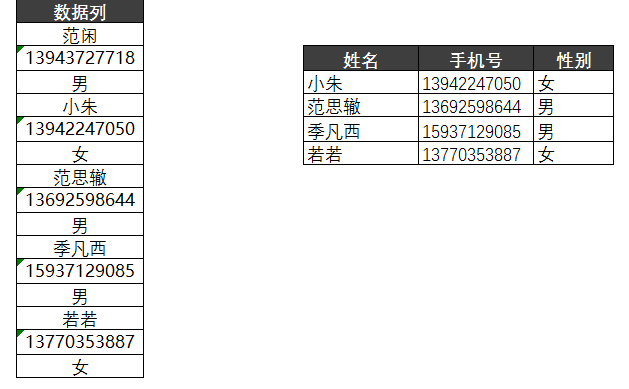# No more Ctrl+V, two tricks to easily convert one column of data into multiple columns in ExcelHello everyone, I am a Xiaoshuang who lurks in many Excel communication groups and makes a fuss from time to time~

In one of the groups, I saw such a question: How to organize titles and links in batches?

I briefly organized it (as shown in the table below), and the general requirement is: organize the left table into the form of the right table.▲ For the convenience of demonstration, only part of the data is shown

What would you do if it were you?

The friends in the group each expressed their views:

Filter by misplacement? Mobile phone numbers all start with 1, this method works!With the help of cell references? This method works!The above methods are all very good methods.

The only disadvantage is: once we add or modify the content of the data source, all operations need to be performed again.

So this article shares two methods with you, the purpose is to dynamically update the data when adding or modifying the data source, let’s take a look with me~

❶ Index function index method

❷ PowerQuery M function method

## 1. Index function index method

Let’s take a look at the specific operation first~

As shown in the figure below, enter the following formula in cell E3:

`= INDEX ( \$B\$2 : \$B\$11 , ROW ( A1 )* 2 - 1 )`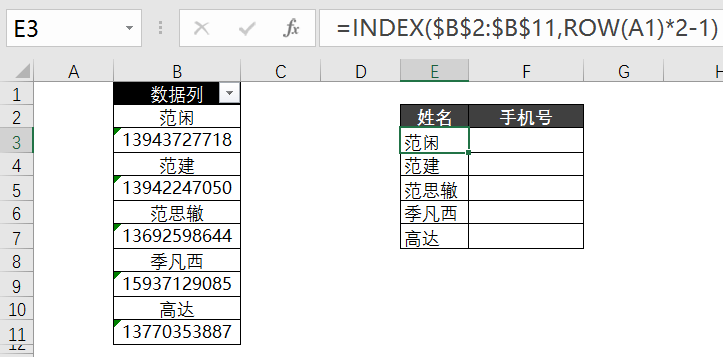Enter the following formula in cell F3:

`= INDEX ( \$B\$2 : \$B\$11 , ROW ( A1 )* 2 )`Just two function formulas and you’re done!

Let’s briefly talk about the principle of the formula~

INDEX function description: The INDEX function can return the cell reference at the intersection of the specified row and column.

=INDEX(area,rows,[columns],[areas])

The formula in the case is as follows:

`= INDEX ( \$B\$2 : \$B\$11 , ROW ( A1 )* 2 - 1 )`

In the formula, the data range is \$B\$2:\$B\$11, and the name column is in the position of 1, 3, 5, … in the green area.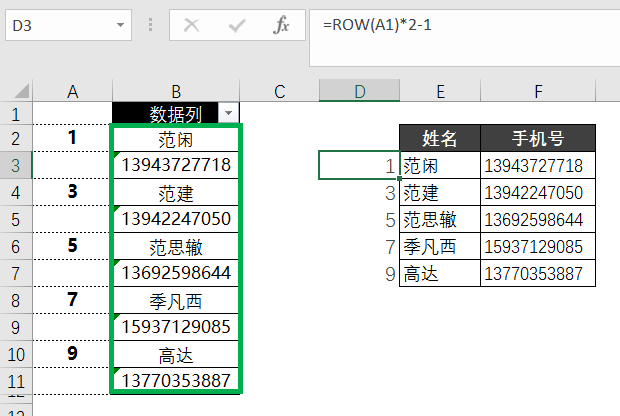So we need to construct an arithmetic sequence 1, 3, 5…, the corresponding formula is as follows:

`= ROW ( A1 )* 2 - 1`

ROW (A1) indicates that the number of rows is 1, and ROW (A1)*2-1=1*2-1=1.

ROW (A2) means that the number of rows is 2, ROW (A2)*2-1=2*2-1=3.

ROW (A3) means that the number of rows is 3, ROW (A3)*2-1=3*2-1=5.

Similarly, if we want to get the mobile phone number, we need to construct an arithmetic sequence 2, 4, 6…

The arithmetic difference formula is = ROW (A1)*2, so the whole function is:

`= INDEX ( \$B\$2 : \$B\$11 , ROW ( A1 )* 2 )`

👉 Summary of ideas:

Use the Row function to construct the arithmetic sequence 1, 3, 5…, use the Index function to index to get the name column, use the Row function to construct the arithmetic sequence 2, 4, 6…, use the Index function to index to get the mobile phone Number.

So far, the method of the Index function has been introduced. Next, let’s introduce a PowerQuery M function method. Be patient and read on!

## 2. PowerQuery M function method

Let’s take a look at the specific steps:

▋Step 1: Import the data source into the PQ editor

Select the table area – select the [Data] tab – [From Table/Area] – the Create Table dialog box appears, press the [OK] button.

At this point, the table will be imported into the PQ editor~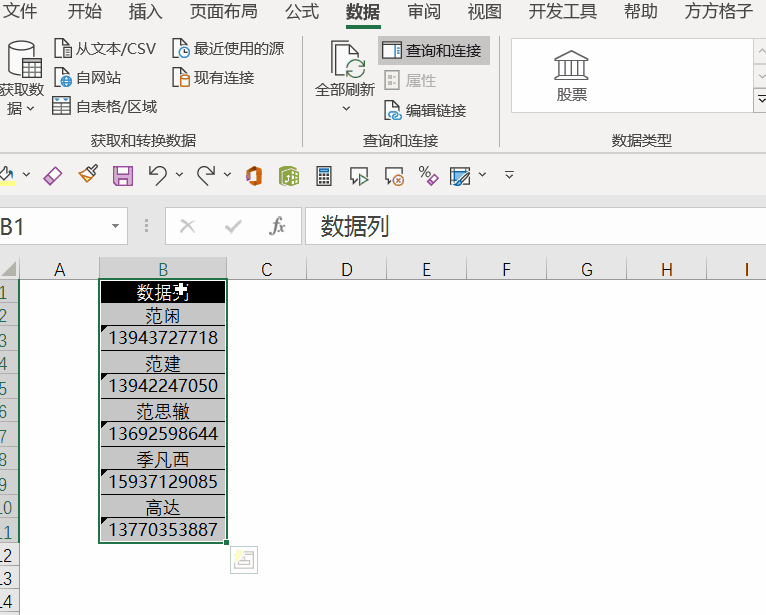▋Step 2: Deepen the data column into a list and select the data column

Right-click the data column – select [Deepening] or select Convert to List] under the [Conversion] tab ;

PS: The purpose of deepening and converting to a list is to extract a certain column of data in the current table to form a list, which is convenient for subsequent operations.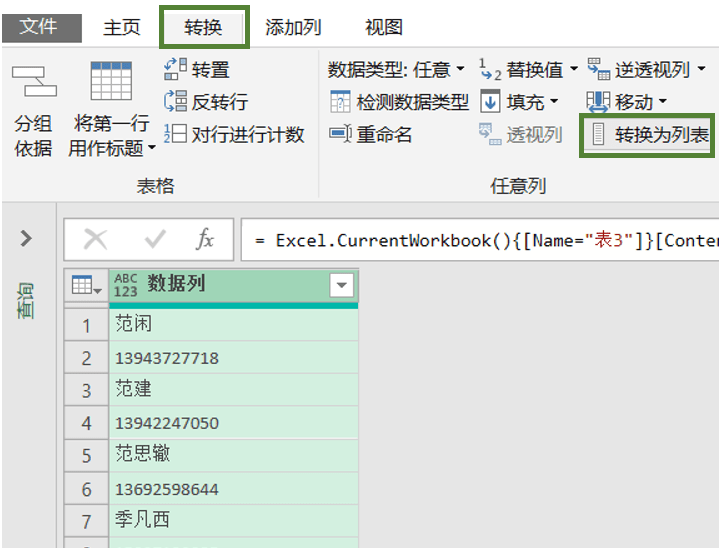Now it becomes a list↓↓↓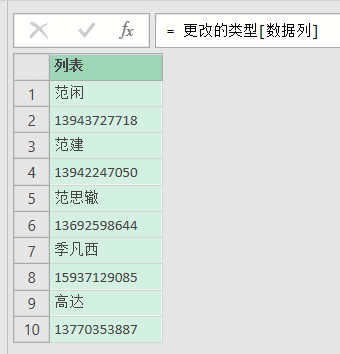▋Step 3: Disassemble every two pieces of information into a List

Click, fx to add a formula in the right edit bar, the formula is as follows:

`= List.Split data column 2 ) _ `At this point, the data is split into two elements and one piece of information~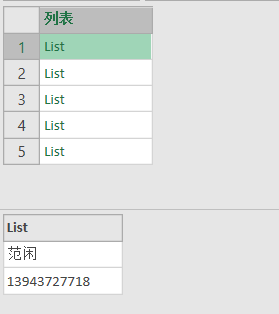▋Step 4: Convert the split List into a Table

This step uses the Table.FromList function.

The formula is as follows:

`= Table . FromList (custom 1 , each _ ,{ "name" , "phone number" })  `

Operation animation: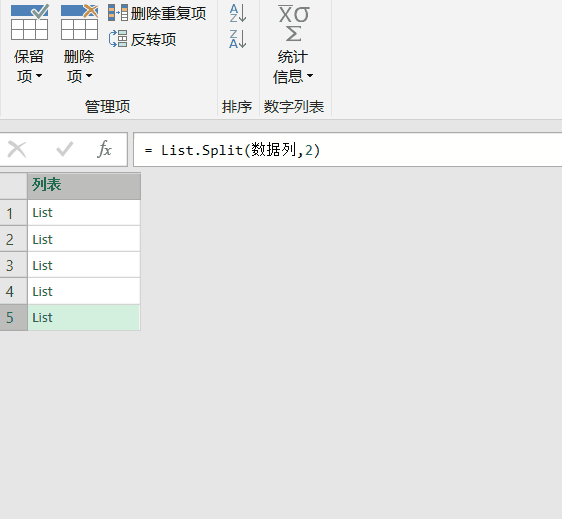▋Step 5: Real-time update

Using the PQ approach, we can update in real time~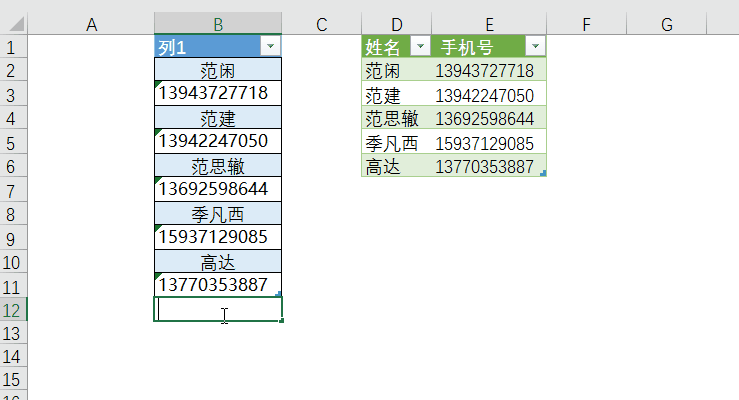Let us briefly introduce the M function involved in the case below.

❶ List.Split: list splitting

`= List . Split (list, each split a few)`

Split means to separate, and List.Split means to split the list every N to form a separate List.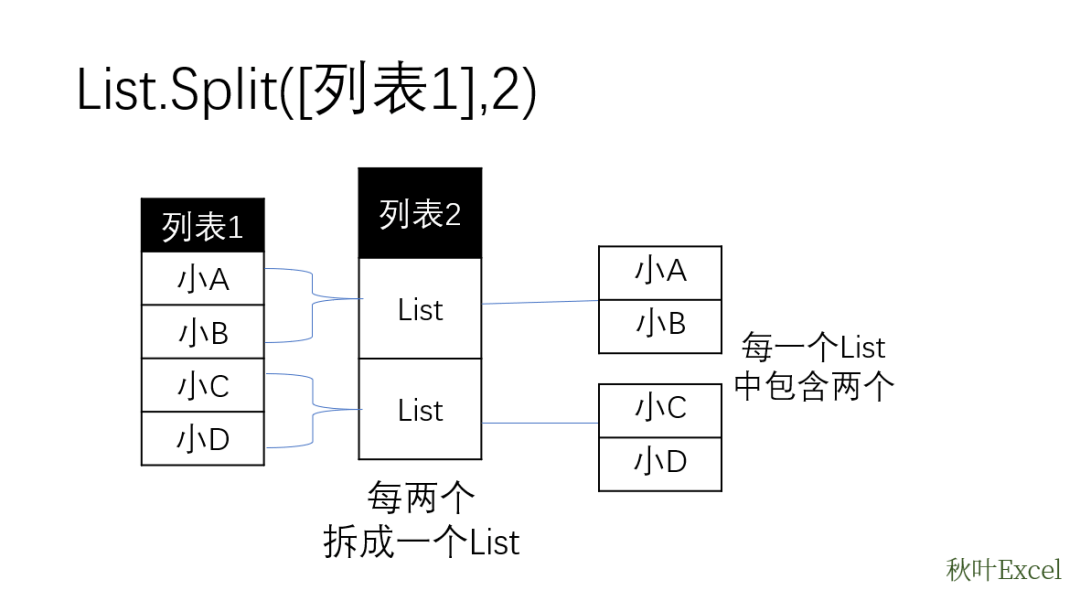READ ALSO: Apple Music now available in the Microsoft Windows 11 app store

Our data is a complete information every 2 elements, so we need to use the List.Split function to split.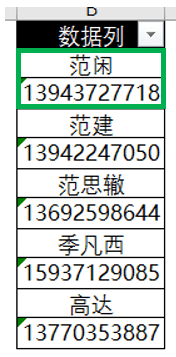❷ Table.FromList: Convert from list to table

=Table.FromList( list, optional how to handle list as list , optional – corresponding header, optional – default value for null, optional – how to handle extra values)

After splitting, we need to convert the List into a Table, so we need to use the Table.FromList function.

`= Table . FromList (custom 1 , each _ ,{ "name" , "phone number" })  `

The first parameter: it is a List, where the custom 1 is the column split by List.Split in the previous step.

The second parameter: For the operation of the previous step, since there is nothing to be processed here, write each _ directly.

The third parameter: the column name after returning the table, the column name is {“name”, “mobile phone number”}.

👉 Idea summary:

The data column in the table is deepened into a list because every 2 elements is a piece of information, so you can use the List.Split function to split every 2 elements into a List.

Finally, use the Table.FromList function to convert the split List into a Table.

At this point, the practice of the M function is over~

## 3. Summarize

❶ Index function The most common method of indexing, which is one of the classic uses of the Index function, is indexed by constructing regular index values.

❷ The method of M function of PQ needs careful study.

Use deepening to extract a column of data in the query table to form a list; use the List.Split function to split the list so that every 2 elements form a list; use Table.FromList to convert the List into a Table.

These two methods can dynamically update data when adding or modifying data sources !

test you:

In the case, every two elements are used as one piece of information. If there are three elements, what would you do?